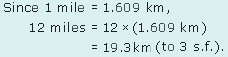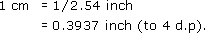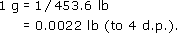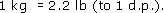Language, notation and formulas

Start this free course now. Just create an account and sign in. Enrol and complete the course for a free statement of participation or digital badge if available.

Free course

# 3.4.1 Try some yourself

## Activity 18

1 Use the formula 1 mile = 1.609 km to find 12 miles in kilometres rounding your answer to 3 significant figures.## Activity 19

Turn the following formulas round to obtain centimetres in terms of inches, and grams in terms of pounds giving your answer to 4 decimal places:

1 inch = 2.54 cm;

1 Ib = 453.6 g (to 4 s.f.).

How many pounds are there in a kilogram?

From 2.54 cm = 1 inch, it follows thatFrom 453.6 g = 1 lb, it follows thatSince 1 kg is 1000 g,## Activity 20

You sell lawn-edging strip by the metre, but some customers have measured their lawns in feet so you have to convert their measurements into metres.

You know the perimeter of a rectangular lawn is:

perimeter = 2 × length + 2 × breadth.

Check whether it makes a difference if you convert to metres before or after you apply the formula.

• (a) Use 1 ft = 0.3048 m to convert the measurements of a lawn that is 12.5 ft by 10 ft into metres. Then use the formula given above to find the perimeter of the lawn in metres.

• (b) Use the formula given above to find the perimeter of the lawn in feet, and then convert this into metres.

• (a) As 1 ft = 0.3048 m, it follows that

•     12.5 ft = 12.5 × 0.3048 m = 3.810 m

• and

•     10 ft = 10 × 0.3048 m = 3.048 m.

• Therefore the lawn is 3.810 m by 3.048 m.

• From the formula for the perimeter,

•     perimeter = (2 × 3.810 m) + (2 × 3.048 m) = 13.716 m.

• So the perimeter of the lawn in metres is 13.716 m.

• (b) From the formula for the perimeter,

•     perimeter = (2 × 12.5 ft) + (2 × 10 ft) = 45 ft.

• So the perimeter of the lawn in feet is 45 ft.

• To convert this into metres, use 1 ft = 0.3048 m, then

•     45 ft = 45 × 0.3048 m = 13.716 m.

• This is the same answer for the perimeter as in part (a). However, the method in part (b) is quicker and, as it involves fewer calculations, it affords less opportunity for making mistakes.

• A further point to bear in mind is that the perimeter formula requires consistent units – you cannot, for instance, use length measured in feet and breadth measured in metres.

MU120_4M6